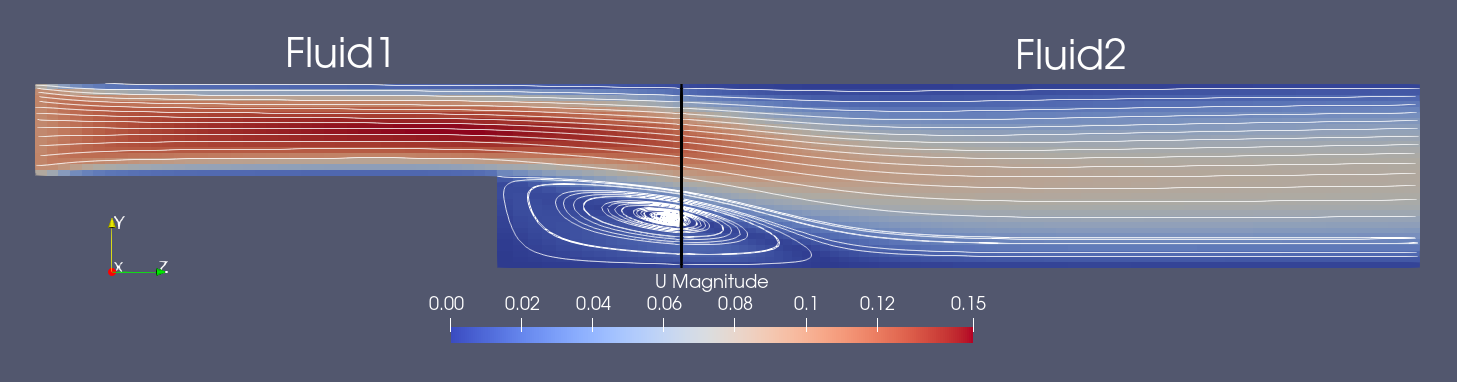This tutorial describes how to run a partitioned fluid simulation using the preCICE OpenFOAM adapter with its custom boundary conditions.

## Setup

This scenario consists of two incompressible fluid solvers in series. The case is a two-dimensional flow over a backwards facing step. Fluid1 consists of an incoming channel that opens into a bigger one over a sudden step. Fluid2 is the remaining part of the bigger channel, where the flow develops behind the step. The fluid will develop a recirculation region behind the step which will also expand across the coupling interface

The key point of this tutorial is the demonstration of custom inlet-outlet OpenFOAM boundary conditions for velocity and pressure. These can dynamically switch their behavior according to the underlying flow direction. The boundary conditions coupledVelocity and coupledPressure come with the preCICE OpenFOAM adapter and can be set in the corresponding start time dictionaries for velocity and pressure.

## Running the Simulation

Open two separate terminals and start the desired fluid1 and fluid2 participants by calling the respective run script. For example:

cd fluid1-openfoam
./run.sh


and

cd fluid2-openfoam
./run.sh


## Post-processing

The OpenFOAM solvers generate a .foam file each. You can open this file in ParaView. An example of the visualized expected results at $t=3.0s$ looks as follows:We observe that the velocity and pressure values are smooth across the interface. Going through different time steps, we can also see how our inlet-outlet boundary conditions accurately changed behavior when the recirculation travelled inside the coupling interface.

Plotting the velocity in z-direction over the coupling interface at $t=2.5s$ shows a smooth transition from negative to positive values: